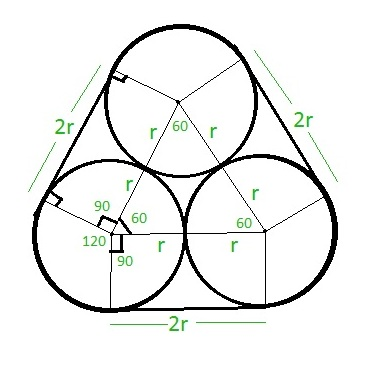Related Articles

# Length of rope tied around three equal circles touching each other

• Last Updated : 06 Jun, 2021

Given r is the radius of three equal circles touching each other. The task is to find the length of the rope tied around the circles as shown below:Examples:

Input: r = 7
Output: 86

Input: r = 14
Output: 172

Approach: As it can be clearly seen from above image, the part of the length of rope which is not touching the circle is 2r + 2r + 2r = 6r
The part of the rope which is touching the circles make a sector of 120 degrees on each circle. Thus, three sectors of 120 degrees each can be considered as a complete one circle of 360 degrees.
Therefore, Length of rope touching the circle is 2 * PI * r where PI = 22 / 7 and r is the radius of the circle.
Hence, the total length of the rope will be ( 2 * PI * r ) + 6r.

Below is the implementation of the above approach:

## C++

 `// C++ program to find the length``// of rope``#include``using` `namespace` `std;``#define PI 3.14159265` `// Function to find the length``// of rope``float` `length_rope( ``float` `r )``{``    ``return` `( ( 2 * PI * r ) + 6 * r );``}` `// Driver code``int` `main()``{``    ``float` `r = 7;``    ``cout<<``ceil``(length_rope( r ))<

## C

 `// C program to find the length``// of rope``#include ``#define PI 3.14159265` `// Function to find the length``// of rope``float` `length_rope( ``float` `r )``{``    ``return` `( ( 2 * PI * r ) + 6 * r );``}` `// Driver code``int` `main()``{``    ``float` `r = 7;``    ``printf``(``"%f"``,``           ``length_rope( r ));``    ``return` `0;``}`

## Java

 `// Java code to find the length``// of rope``import` `java.lang.*;` `class` `GFG {` `    ``static` `double` `PI = ``3.14159265``;` `    ``// Function to find the length``    ``// of rope``    ``public` `static` `double` `length_rope(``double` `r)``    ``{``        ``return` `((``2` `* PI * r) + ``6` `* r);``    ``}` `    ``// Driver code``    ``public` `static` `void` `main(String[] args)``    ``{``        ``double` `r = ``7``;``        ``System.out.println(length_rope(r));``    ``}``}`

## Python3

 `# Python3 code to find the length``# of rope``PI ``=` `3.14159265``    ` `# Function to find the length``# of rope``def` `length_rope( r ):``    ``return` `( ( ``2` `*` `PI ``*` `r ) ``+` `6` `*` `r )``    ` `# Driver code``r ``=` `7``print``( length_rope( r ))`

## C#

 `// C# code to find the length``// of rope``using` `System;` `class` `GFG {``    ``static` `double` `PI = 3.14159265;` `    ``// Function to find the length``    ``// of rope``    ``public` `static` `double` `length_rope(``double` `r)``    ``{``        ``return` `((2 * PI * r) + 6 * r);``    ``}` `    ``// Driver code``    ``public` `static` `void` `Main()``    ``{``        ``double` `r = 7.0;``        ``Console.Write(length_rope(r));``    ``}``}`

## PHP

 ``

## Javascript

 ``
Output:
`86`

Attention reader! Don’t stop learning now. Get hold of all the important DSA concepts with the DSA Self Paced Course at a student-friendly price and become industry ready.  To complete your preparation from learning a language to DS Algo and many more,  please refer Complete Interview Preparation Course.

In case you wish to attend live classes with experts, please refer DSA Live Classes for Working Professionals and Competitive Programming Live for Students.

My Personal Notes arrow_drop_up